4174.0 - Spectator Attendance at Sporting Events, 2009-10
ARCHIVED ISSUE Released at 11:30 AM (CANBERRA TIME) 21/12/2010  Final
 Page tools: .mffeedback,#pjs { display :none; } Enable Javascript to Print PagesPrint All Explanatory Notes Glossary Abbreviations Quality Declaration - Summary Data quality (Technical Note) Data Source TECHNICAL NOTE DATA QUALITY RELIABILITY OF THE ESTIMATE 1 Since the estimates in this publication are based on information obtained from a sample of persons, they are subject to sampling variability. That is, they may differ from those that would have been produced had all persons been included in the survey. 2 One measure of the likely difference is given by the standard error (SE), which indicates the extent to which an estimate might have varied by chance because only a sample of persons was included. There are about 2 chances in 3 (67%) that the sample estimate will differ by less than one SE from the number that would have been obtained if all persons had been surveyed, and about 19 chances in 20 (95%) that the difference will be less than two SEs. 3 Another measure of the likely difference is the relative standard error (RSE), which is obtained by expressing the SE as a percentage of the estimate.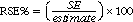4 RSEs for all estimates in the publication are available free-of-charge on the ABS website released in spreadsheet format as an attachment to this publication. 5 In the tables in this publication, only estimates (numbers or percentages) with RSEs less than 25% are considered sufficiently reliable for most purposes. However, estimates with larger RSEs have been included and are preceded by an asterisk (e.g. *3.4) to indicate they are subject to high SEs and should be used with caution. Estimates with RSEs greater than 50% are preceded by a double asterisk (e.g. **2.1) to indicate that they are considered too unreliable for general use. CALCULATION OF STANDARD ERRORS 6 Standard errors can be calculated using the estimates (counts or percentages) and the corresponding RSEs. For example, Table 3 shows the estimated number of persons (aged 15 years or more) who attended basketball in the 12 months before interview, which is 220,800. The corresponding RSE table available on the ABS website shows the RSE for this estimate is 8.7%. The SE is calculated by: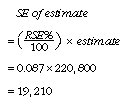7 Therefore, there are about two chances in three that the value that would have been produced if all dwellings had been included in the survey will fall within the range 201,600 and 240,000 and about 19 chances in 20 that the value will fall within the range 182,400 to 259,200. This example is illustrated in the diagram below.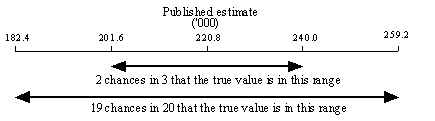PROPORTIONS AND PERCENTAGES 8 Proportions and percentages formed from the ratio of two estimates are also subject to sampling errors. The size of the error depends on the accuracy of both the numerator and the denominator. A formula to approximate the RSE of a proportion is given below. The formula is only valid when x is a subset of y: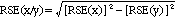9 Consider the example given above of the number of persons who attended basketball (220,800). Of these, 40% (or approximately 88,300) attended once or twice in the 12 months before interview (Table 6). As already noted, the SE of 220,800 is approximately 19,210 which equates to an RSE of about 8.7%. The SE and RSE of 88,300 are approximately 13,333 and 15.1% respectively. Applying the formula above, the estimate of 40% will have an RSE of: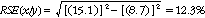10 This gives a SE for the proportion (40%) of approximately 5 percentage points. Therefore, if all persons had been included in the survey, there are 2 chances in 3 that the proportion that would have been obtained is between 35% to 40% and about 19 chances in 20 that the proportion is within the range 30% to 50%. DIFFERENCES 11 Published estimates may also be used to calculate the difference between two survey estimates (of counts or percentages). Such an estimate is subject to sampling error. The sampling error of the difference between two estimates depends on their SEs and the relationship (correlation) between them. An approximate SE of the difference between two estimates (x-y) may be calculated by the following formula: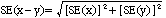SIGNIFICANCE TESTING 12 A statistical significance test for any of the comparisons between estimates can be performed to determine whether it is likely that there is a difference between the corresponding population characteristics. The standard error of the difference between two corresponding estimates (x and y) can be calculated using the formula in paragraph X. This standard error is then used to calculate the following test statistic: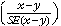13 If the absolute value of this test statistic is greater than 1.96 then there is evidence of a statistically significant difference (at the 5% level) in the two estimates with respect to that characteristic. This statistic corresponds to a 95% confidence interval of the difference. Otherwise, it cannot be stated with confidence that there is a real difference between the population with respect to that characteristic. 14 Tables which show estimates from 2005-06 and 2009-10 have been tested to determine whether changes over time are statistically significant. Significant differences have been annotated. In all other tables which do not show the results of significance testing, users should take account of RSEs when comparing estimates for different populations. 15 The imprecision due to sampling variability, labelled sampling error should not be confused with non-sampling error. Non-sampling error may occur in any collection, whether it is based on a sample or a full count such as a census. Sources of non-sampling error include non-response, errors in reporting by respondents or recording answers by interviewers and errors in coding and processing data. Every effort was made to reduce the non-sampling error by careful design and testing of the questionnaire, training and supervision of interviewers, extensive editing and quality control procedures at all stages of data processing. RELATIVE STANDARD ERRORS 16 Limited space does not allow the SEs and/or RSEs of all the estimates to be shown in this publication. However, RSEs for all tables are available free-of-charge on the ABS website , available in spreadsheet format as an attachment to this publication. Document Selection These documents will be presented in a new window.